• 教学课件 课件PPT 医学培训课件 教育资源 教材 讲义
• 若有两个一元函数 f(x)f(x)f(x) 和 g(x)g(x)g(x) ，我们可以把 ggg 的函数值作为 fff 的自变量，得到一个新的函数称为 f(x)f(x)f(x) 和 g(x)g(x)g(x) 的复合函数，记为 f[g(x)]f[g(x)]f[g(x)]。 如果我们已知上述两...
定义
若有两个一元函数

f

(

x

)

f(x)

和

g

(

x

)

g(x)

，我们可以把

g

g

的函数值作为

f

f

的自变量，得到一个新的函数称为

f

(

x

)

f(x)

和

g

(

x

)

g(x)

的复合函数，记为

f

[

g

(

x

)

]

f[g(x)]

。
如果我们已知上述两个函数

f

(

x

)

f(x)

和

g

(

x

)

g(x)

的导函数

f

′

(

x

)

f^{\prime}(x)

和

g

′

(

x

)

g^{\prime}(x)

，那么我们可以通过以下公式求复合函数

f

[

g

(

x

)

]

f[g(x)]

的导数。

f

[

g

(

x

)

]

′

=

f

′

[

g

(

x

)

]

g

′

(

x

)

f[g(x)]^{\prime}=f^{\prime}[g(x)] g^{\prime}(x)

多重复合函数
以

f

[

g

(

h

(

x

)

)

]

f[g(h(x))]

为例，要对多重复合函数

f

[

g

(

h

(

x

)

)

]

f[g(h(x))]

求导，可以先对

g

[

h

(

x

)

]

g[h(x)]

求导得

g

′

[

h

(

x

)

]

h

′

(

x

)

g^{\prime}[h(x)] h^{\prime}(x)

，再得到

f

[

g

(

h

(

x

)

)

]

′

=

f

′

[

g

(

h

(

x

)

)

]

g

′

[

h

(

x

)

]

h

′

(

x

)

f[g(h(x))]^{\prime}=f^{\prime}[g(h(x))] g^{\prime}[h(x)] h^{\prime}(x)

其它任意多重的复合函数求导同理可得。
链式法则
至于复合函数的求导公式为什么称为链式法则，原因是如果把表示过程用导数的另外一种符号表示如下:

d

y

=

d

y

d

u

d

u

=

d

y

d

u

d

u

d

x

d

x

\mathrm{d} y=\frac{\mathrm{d} y}{\mathrm{~d} u} \mathrm{~d} u=\frac{\mathrm{d} y}{\mathrm{~d} u} \frac{\mathrm{d} u}{\mathrm{~d} x} \mathrm{~d} x

得到：

d

y

d

x

=

d

y

d

u

d

u

d

x

\frac{\mathrm{d} y}{\mathrm{~d} x}=\frac{\mathrm{d} y}{\mathrm{~d} u} \frac{\mathrm{d} u}{\mathrm{~d} x}

举例
求函数

f

(

x

)

=

(

x

2

+

1

)

3

f(x)=\left(x^{2}+1\right)^{3}

的导数。
可设

g

(

x

)

=

x

2

+

1

,

h

(

g

)

=

g

3

g(x)=x^{2}+1, h(g)=g^{3}

，那么则有

h

(

g

(

x

)

)

=

g

(

x

)

3

h(g(x))=g(x)^{3}

。
则求解过程为，

f

′

(

x

)

=

[

h

(

g

(

x

)

)

]

′

=

h

′

(

g

(

x

)

)

g

′

(

x

)

=

3

(

g

(

x

)

)

2

(

2

x

)

=

3

(

x

2

+

1

)

2

(

2

x

)

=

6

x

(

x

2

+

1

)

2

f^{\prime}(x)=[h(g(x))]^{\prime}=h^{\prime}(g(x)) g^{\prime}(x)=3(g(x))^{2}(2 x)=3\left(x^{2}+1\right)^{2}(2 x)=6 x\left(x^{2}+1\right)^{2}


展开全文链式法则
• uuu 和 vvv 都是关于自变量 xxx 的函数。 加减形式： [u±v]′=u′±v′ \left[ u \pm v \right]' = u' \pm v' [u±v]′=u′±v′ 相乘形式： [uv]′=u′v+uv′ \left[ u v \right]' = u' v + uv' [uv]′=u′v+uv′ ...


u

u

和

v

v

都是关于自变量

x

x

的函数。
加减形式：

(

u

±

v

)

′

=

u

′

±

v

′

\bm{ \left( u \pm v \right)' = u' \pm v' }

相乘形式：

(

u

v

)

′

=

u

′

v

+

u

v

′

\bm{ \left( u v \right)' = u' v + uv' }

相除形式：

(

u

v

)

′

=

u

′

v

−

u

v

′

v

2

\bm{ \left( \frac{u}{v} \right)' = \frac{u'v - uv'}{v^2} }


展开全文高等数学
• 1、复合函数求导 复合函数就是多个函数把它嵌套起来。复合函数关键理解就是：内层函数的输出是外层函数的输入。 复合函数的求导法则：链式法则 因为复合函数是一层一层的由内向外的复合而得；那么复合函数的...
1、复合函数求导
复合函数就是多个函数把它嵌套起来。复合函数关键理解就是：内层函数的输出是外层函数的输入。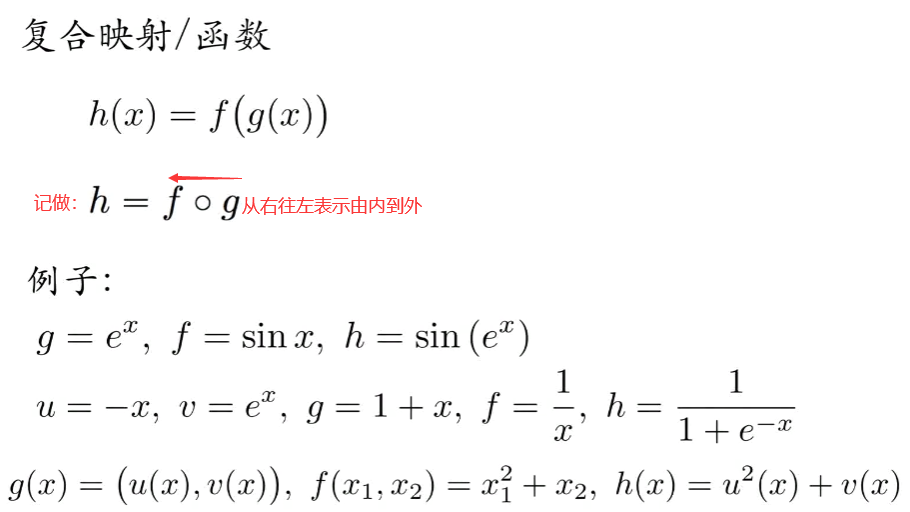复合函数的求导法则：链式法则
因为复合函数是一层一层的由内向外的复合而得；那么复合函数的求导链式法则就是由外层向内层逐步的求导，即一层一层的求下去。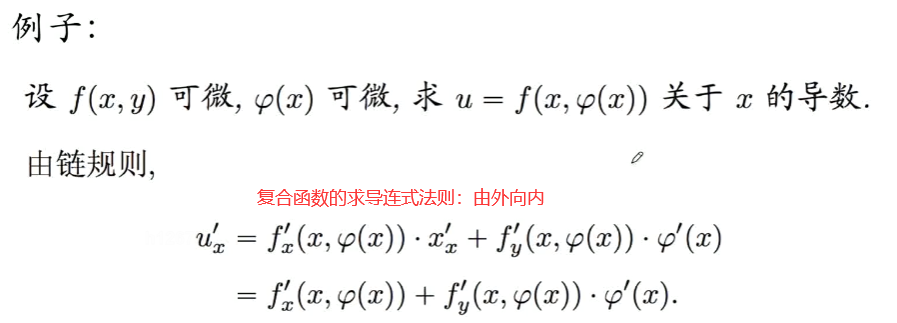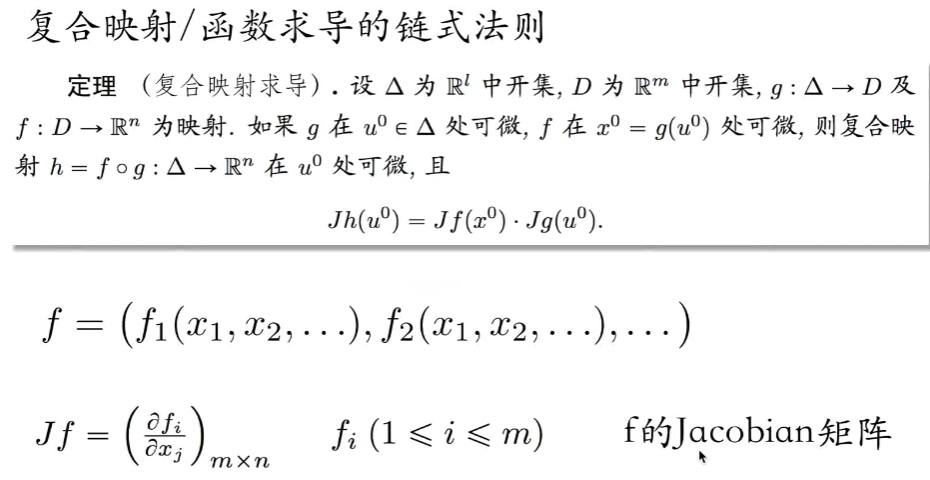对于一般的映射可以理解成输出是一个向量，这个映射满足  其中是的雅克比矩阵，这个矩阵的第一行第一列呢因为是一个映射，这个第一行第一列是关于求偏导。第一行第二列是关于求偏导。第二行第一列就是关于求偏导，第二行第二列就是关于求偏导。如果有了复合函数h的雅克比矩阵，其实是拿到了这个映射h的整个的复合函数求导。整个复合函数的求导等于的雅克比矩阵乘以的雅克比矩阵。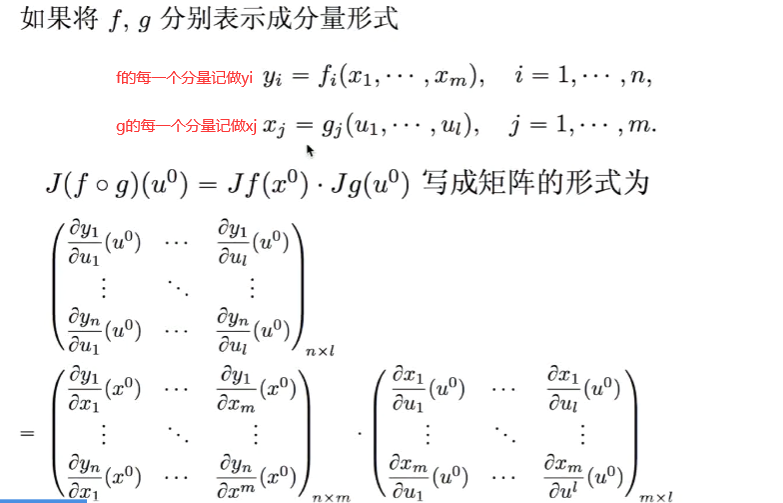由外向内在求导，第一层是y关于求偏导，内部一层就是关于在求偏导。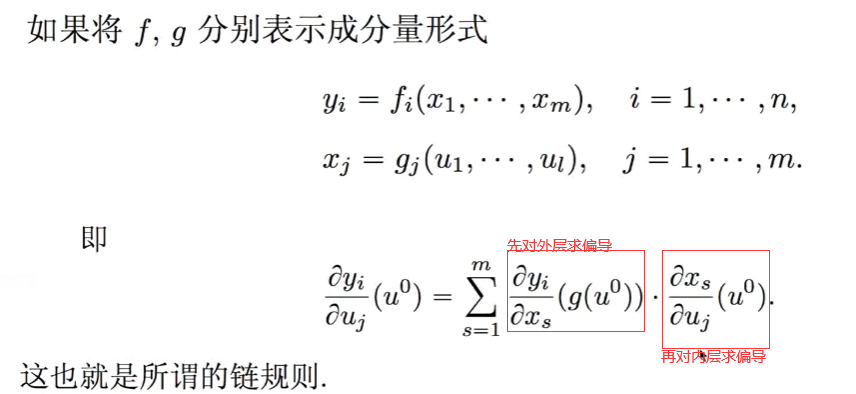Jacobian Matrix(雅可比矩阵)：
在向量微积分中，雅可比矩阵是一阶偏导数以一定方式排列成的矩阵，其行列式称为雅可比行列式。雅可比矩阵的重要性在于它体现了一个可微方程与给出点的最优线性逼近。因此，雅可比矩阵类似于多元函数的导数。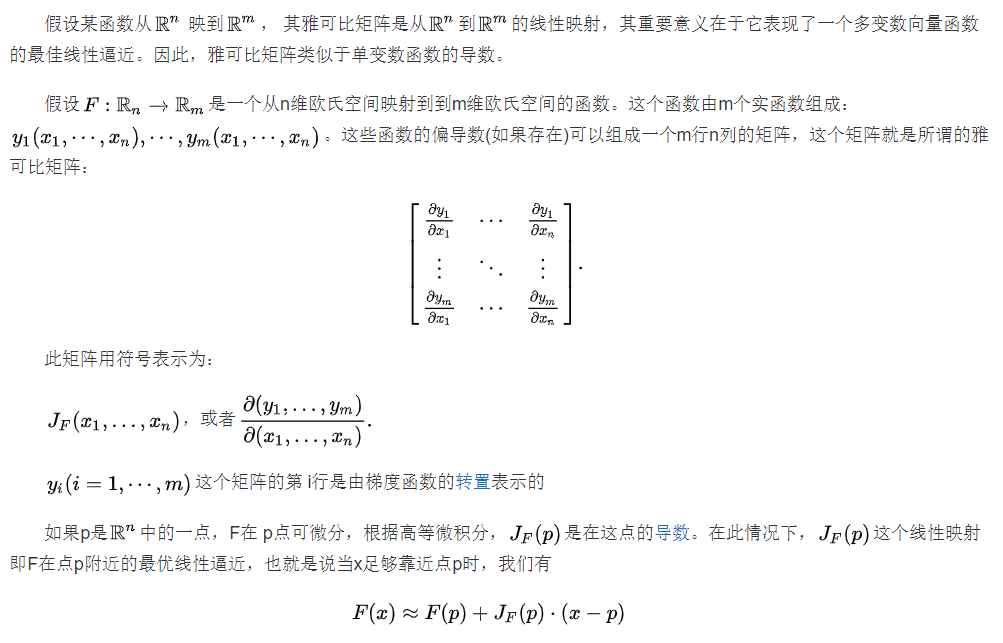具体查看：https://wenku.baidu.com/view/4707bfd176a20029bd642d8c.html
2、重积分：连续的分割求和取极限。
一元积分(一重积分)是想求的曲边梯形的面积。即分割求和取极限(把它分割成一个个的小矩形，让这些矩形的宽度趋于0，算这些矩形的面积)
二重积分：求体积(曲面下曲边柱体的体积)(先求曲线下每一小块的面积，再求每一小块对应的高度，最后求体积；思想：分割求和取极限)。
三重积分：（分割定义域，分割成一小块一小块的，每一小块的体积叫做，每一小块的体积乘上这一小块的位置所处的函数值，乘完之后后，求和取极限这个就是三重积分。）
三重积分可视化展示的话需要的是4个轴，画4维物体。分别是X，Y，Z，f。相当于在四维空间中画一个三维的物体。
三重积分的应用，有一个铁块，知道每一小块的密度之后，求铁块的质量，f就是密度，他随着每一小块的位置不同密度不一样，想要计算这个铁块的质量就得使用三重积分。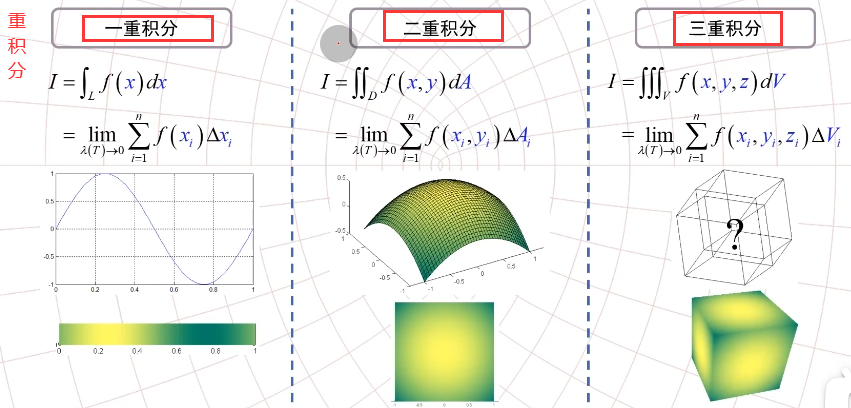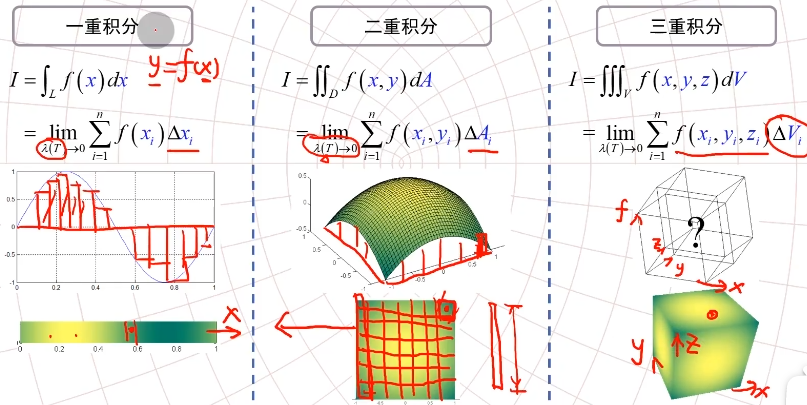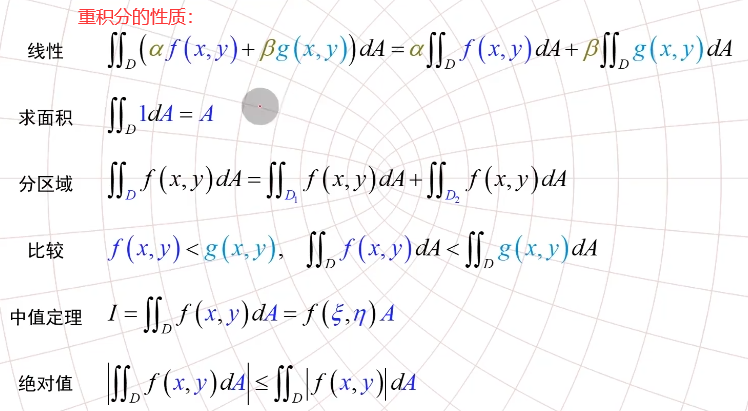二重积分可以算面积，三重积分可以算体积，如何算体积呢？答：对这个区域做积分，被积的函数等于1。如下：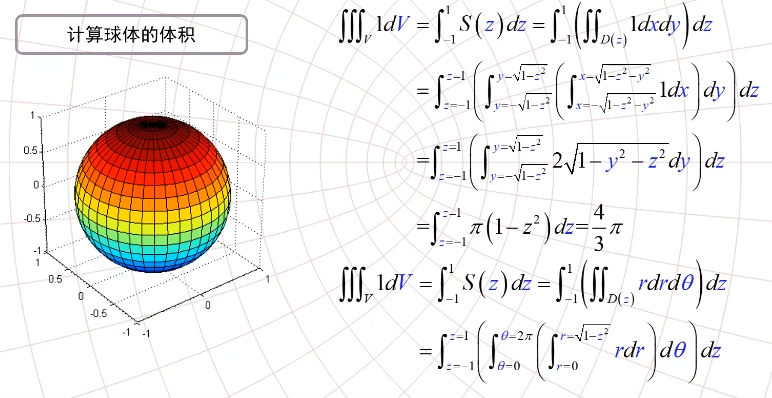在直角坐标系下;dv=dxdydz；在极坐标系下：dv=rdrdθdz
3、偏导数与全微分

微分：局部线性化。求微分就是局部线性化。

如果一个函数他是可以微分的，这就说明这个函数在局部(这个小的局部放大的看就是近似一个平面，平面的方程是，假设我们取的点是那么在这个点附近我们可以使用平面来代替，就变成了一个局部坐标系，之后让都趋近与0，把写成，这个函数的全微分就是其中A和B就是平面方程的两个系数，此时其实是和的函数，而且是一个线性函数，因为是一个平面，要求A的话就让等于0，对求导，也就是偏z和偏x，就是偏导；B同理。)
一元函数的话，局部线性化之后就是一个一次函数；如果是二元函数的话，局部线性化之后就是一个平面。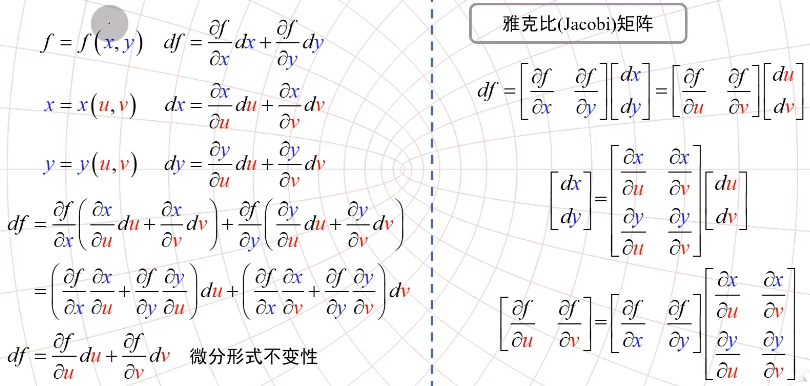一元函数的导数他是一个数，多元函数的导数他是一个矩阵，叫做雅克比矩阵。

雅克比矩阵描述的是说：在这一点局部的坐标变换最接近于哪一个线性变换，这就是雅克比矩阵的几何意义。

雅克比矩阵行列式。雅克比矩阵的行列式就叫雅克比值。

https://www.bilibili.com/video/BV11T4y1w739?from=search&seid=12710661420161959659
https://www.bilibili.com/video/BV1NJ411r7ja?from=search&seid=12710661420161959659
重积分换元一定要乘以一个雅克比，来保证他们的面积是相同的。类比于一元积分换元时要多乘以一个导数。这个导数确实就是雅克比矩阵行列式（1x1矩阵的行列式就是这个数字本身了）。
极坐标与直角坐标：极坐标就是一个圈一个圈的跟雷达似的。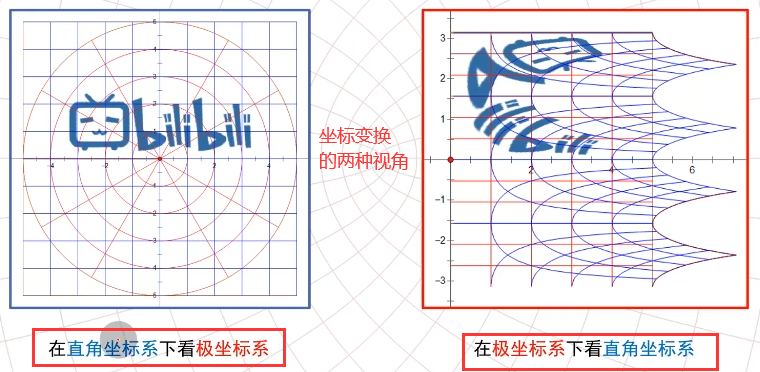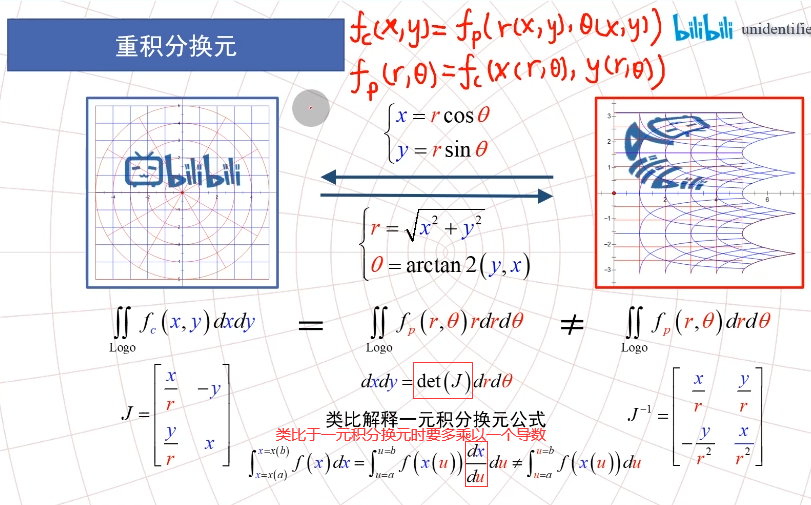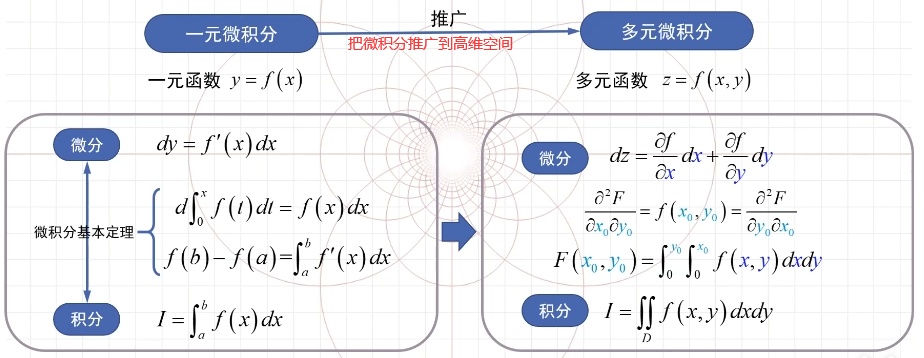PS：多元函数微分的宗旨就是局部线性化，求全微分。输出量就是输入量的线性组合，组合的系数就是求偏导数。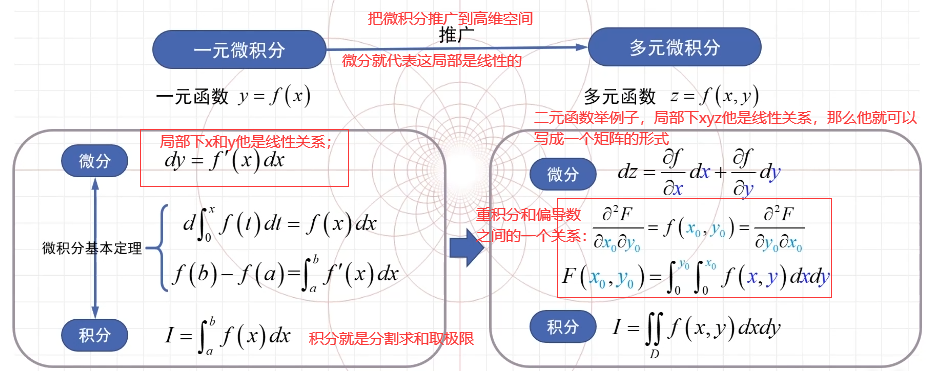展开全文• 1.提示 从导数的定义出发 2证明 参考：传送门 3.总结 任何公式、定理、法则等都是从它最简单的定义推起
1.提示
从导数的定义出发
2证明参考：传送门
3.总结
任何公式、定理、法则等都是从它最简单的定义推起
展开全文• 1 ，例子 ：匀变速运动的瞬时速度 物理公式 ： 2 ，导数的几何意义 ： ...5 ，复合函数求导 ： 定义 ：两个函数的导数的乘积 例子 ： 例子 ： 6 ，高阶导数 ： 高阶导数 ： 求导再求导 例如 ： 2 阶导数 ...高等数学
• 若函数u=ϕ(t)、v=ψ(t)u=ϕ(t)、v=ψ(t)u = \phi(t)、v = \psi(t)都在点ttt可导，函数z=f(u,v)z=f(u,v)z=f(u,v)在对应点(u,v)(u,v)(u,v)具有连续偏导数，那么复合函数z=f[ϕ(t),ψ(t)]z=f[ϕ(t),ψ(t)]z=f[\phi(t),...高等数学
• 复合函数求导与隐函数求导习题课PPT学习教案.pptx
• ## 复合函数求导

千次阅读 2017-04-25 16:14:51
https://wenku.baidu.com/view/441521ba69dc5022aaea00fc.html
• h=compose(g,f) % 函数组合，将f作为g的自变量 i=diff(h)   result f = sin(x) g = x^2 h = sin(x)^2 i = 2*cos(x)*sin(x) >>   resource [文档] ww2.mathworks....
•文档
• 在证明由函数y=f(u)与u=φ(x)构成的复合函数y=f[φ(x)]的求导公式dy/dx.du/dx时，在数学分析教材的证明中都用到当Δu趋于零时的无穷小量α，并需要补充义当Δu=0时，α=0，这对初学者来说是不容易理解的.本文给出的...
• 本文给出两种证明方式，第一种高等数学...设函数和, 其中 x 为自变量，f(g(x))在个g(x)处可导 ,g(x)在x出可导。 根据可导的定义得： 其中当时 （这里的就是高等数学书上说的增量 Δx） 同理： 其中时 现...
•抽象代数
•文档
• 求导公式 导数运输法则 复合函数求导法则 幂指函数求导 取对数后按照符合函数求导法则数学
• emmm想了下这一篇就不做关于一元函数求导的回顾了，一元函数求导显函数、隐函数、复合函数、参数方程函数，要回顾也不是一两句能说清楚的，推荐去看以前的相关内容，在第二章。 多元函数求导的内容也分为显函数、...
• 高等数学电子同济六多元复合函数求导法则PPT课件.pptx
• 教师资格证面试试讲-14《复合函数求导例题》逐字稿.pdf
• 立即学习:https://edu.csdn.net/course/play/26262/326627?utm_source=blogtoedu 函数相乘的导数： 证明： P： 函数相除的导数： Q： A： 链式法则： （LaTeX公式在线编辑器https://www.latexlive.com/）程序员 数学 编程语言 Python 人工智能
• 链式法则是微积分中的求导法则，用以求一个复合函数的导数。 所谓的复合函数，是指以一个函数作为另一个函数的自变量。如设f(x)=3x，g(x)=x+3，g(f(x))就是一个复合函数，并且g′(f(x))=3 链式法则，若h(x)=f(g(x))...
• 指数复合函数求导与欧拉方程： [f(et)]′=f′(et)∗et [f(e^t)]'=f'(e^t)*e^t[f(et)]′=f′(et)∗et xn∗y(n)+P1∗xn−1∗y(n−1)+……+Pn−1∗xn−1∗y′+Pny=f(x)x ^ { n }*y^{(n)}+P_{1}*x ^ { n-1}*y^{(n-1)}+...
• 一、复合函数求导法则 1.1、一元函数与多元函数符合的情形 1.1.1、证明 1.1.2、推广到中间变量多余两个的 1.2、多元函数与多元函数的复合 1.2.1、为什么将dzdx变成∂z∂x\frac {dz} {dx} 变成\frac {\partial ...
• 1.复合函数求导法 2.全微分形式不变性 3.隐函数求导法 一：复合函数求导数与全微分 例题1：具体点先代后求、导数的定义 例题2：全微分 例题3：变上限 例题4:幂函数形式（多元复合） 例题5 例题6 例题7 ......